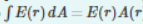# Problem:  Consider a point charge q in three-dimensional space. Symmetry requires the electric field to point directly away from the charge in all directions. To find E(r), the magnitude of the field at distance from the charge, the logical Gaussian surface is a sphere centered at the charge. The electric field is normal to this surface, so the dot product of the electric field and an infinitesimal surface element involves cos(0) = 1. The flux integral is therefore reduced to where E(r) is the magnitude of the electric field on the Gaussian surface, and A(r) is the area of the surface.Determine the magnitude E(r) by applying Gauss's law

###### FREE Expert Solution

Gauss's law:

$\overline{){\mathbf{\oint }}{\mathbf{E}}{\mathbf{·}}{\mathbf{dA}}{\mathbf{=}}{\mathbf{EA}}{\mathbf{=}}\frac{\mathbf{q}}{{\mathbf{\epsilon }}_{\mathbf{0}}}}$

A = 4πr2

86% (415 ratings)###### Problem Details

Consider a point charge q in three-dimensional space. Symmetry requires the electric field to point directly away from the charge in all directions. To find E(r), the magnitude of the field at distance from the charge, the logical Gaussian surface is a sphere centered at the charge. The electric field is normal to this surface, so the dot product of the electric field and an infinitesimal surface element involves cos(0) = 1.

The flux integral is therefore reduced towhere E(r) is the magnitude of the electric field on the Gaussian surface, and A(r) is the area of the surface.

Determine the magnitude E(r) by applying Gauss's law